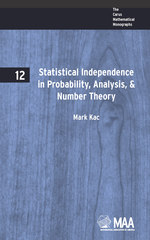# Statistical Independence in Probability, Analysis and Number Theory

MARK KAC
Volume: 12
Edition: 1
Pages: 109
https://www.jstor.org/stable/10.4169/j.ctt5hh8tq

1. Front Matter
(pp. i-viii)
2. PREFACE
(pp. ix-xii)
Mark Kac
(pp. xiii-xiv)
4. CHAPTER 1 FROM VIETA TO THE NOTION OF STATISTICAL INDEPENDENCE
(pp. 1-12)

1. A formula of Vieta. We start from simple trigonometry. Write

$\sin x = 2\sin \frac{x}{2}\cos \frac{x}{2}$

$= {2^2}\sin \frac{x}{4}\cos \frac{x}{4}\cos \frac{x}{2}$

(1.1)$= {2^3}\sin \frac{x}{8}\cos \frac{x}{8}\cos \frac{x}{4}\cos \frac{x}{2}$

.

.

.

$= {2^n}\sin \frac{x}{{{2^n}}}\prod\limits_{k = 1}^n {\cos \frac{x}{{{2^k}}}.}$

From elementary calculus we know that, for$x \ne 0,$

$1 = \mathop {\lim }\limits_{n \to \infty } \frac{{\sin \frac{x}{{{2^n}}}}}{{\frac{x}{{{2^n}}}}} = \frac{1}{x}\mathop {\lim }\limits_{n \to \infty } {2^n}\sin \frac{x}{{{2^n}}},$

and hence

(1.2)$\mathop {\lim }\limits_{n \to \infty } {2^n}\sin \frac{x}{{{2^n}}} = x.$

Combining (1.2) with (1.1), we get

(1.3)$\frac{{\sin x}}{x} = \prod\limits_{k = 1}^\infty {\cos \frac{x}{{{2^k}}}} .$

A special case of (1.3) is of particular interest. Setting$x = \frac{\pi }{2},$we obtain

(1.4)$\frac{2}{\pi } = \prod\limits_{n = 1}^\infty {\cos \frac{\pi }{{{2^{n + 1}}}}}$

$= \frac{{\sqrt 2 }}{2}\frac{{\sqrt {2 + \sqrt 2 } }}{2}\frac{{\sqrt {2 + \sqrt {2 + \sqrt 2 } } }}{2}$...,

a classical formula due to Vieta.

2. Another look at Vieta’s formula. So far everything has been straightforward and familiar.

Now let us take a look a t (1.3) from a different point of view.

It is known that every real number$t,0 \le t \le 1$, can be writtenuniquelyin the form

(2.1)$t = \frac{{{\varepsilon _1}}}{2} + \frac{{{\varepsilon _2}}}{{{2^2}}} + ...,$

where each ε is either 0 or 1....

5. CHAPTER 2 BOREL AND AFTER
(pp. 13-35)

You have all heard that if you play a fair game of chance, then, in the long run, it is unlikely that you will get rich. “The law of averages will take care of it” is what one hears uttered wisely in this and similar connections. What is this “law of averages”? Is it some sort of a physical law, or is it a purely mathematical statement? It is mostly the latter, although the agreement with experimental evidence is remarkably good. Let us forget about experimental evidence and concentrate on the mathamatical issues. Suppose I toss a “fair” coin, winning...

6. CHAPTER 3 THE NORMAL LAW
(pp. 36-52)

In § 1 of Chapter 2, we discussed the “weak law of large numbers.” A more precise result was proved by De Moivre to the effect that

(1.1)$\mathop {\lim }\limits_{n \to \infty } \mu \{ {\omega _1}\sqrt n < {r_1}(t) + \cdots + {r_n}(t) < {\omega _2}\sqrt n \} = \frac{1}{{\sqrt {2\pi } }}\int\limits_{{\omega _1}}^{{\omega _2}} {{e^{ - {y^{1/2}}}}} dy$

The reader will have no trouble interpreting this result in probability terms. An elementary proof can be based on formula (5.6) of Chapter 1, and (1.1) becomes equivalent to the purely combinatorial formula

(1.2)$\mathop {\lim }\limits_{n \to \infty } \sum\limits_{\frac{n}{2} + \frac{{{\omega _1}}}{2}\sqrt n < l < \frac{n}{2} + \frac{{{\omega _2}}}{2}\sqrt n } {\frac{1}{{{2^n}}}\left( {_l^n} \right)} = \frac{1}{{\sqrt {2\pi } }}\int\limits_{{\omega _1}}^{{\omega _2}} {{e^{ - {y^{2/2}}}}} dy.$

Adroit use of Stirling’s formula will yield (1.2), but this proof will also obscure the nature of the theorem. Attempts to generalize (1.1) provided one of the strongest motivations for developing analytical tools of probability theory....

7. CHAPTER 4 PRIMES PLAY A GAME OF CHANCE
(pp. 53-79)

A number theoretic functionf(n)is a function denned on the positive integers 1, 2, 3, · · · . The meanM{f(n)}offis denned as the limit (if it exists)

(1.1)$M\{ f(n)\} = \mathop {\lim }\limits_{N \to \infty } \frac{1}{N}\sum\limits_{n = 1}^N {f(n)}$

IfAis a set of positive integers, we denote byA(N)the number of its elements among the firstNintegers. If

A(N) (1.2)$\mathop {\lim }\limits_{N \to \infty } \frac{{A(N)}}{N} = D\{ A\}$

exists, it is called thedensity of A.The density is analogous to the relative measure (see § 5 of Chapter 3), and like relative measure it is not completely additive. Consider the integers divisible by a primep....

8. CHAPTER 5 FROM KINETIC THEORY TO CONTINUED FRACTIONS
(pp. 80-93)

About the middle of the nineteenth century attempts were begun to unite the disciplines of mechanics and thermodynamics.

The main problem was to derive the Second Law of Thermodynamics from the picture of matter as consisting of particles (atoms or molecules) subject to forces and obeying the laws of mechanics.

In the hands of Maxwell and Boltzmann (and later J. W. Gibbs) this kinetic approach flowered into one of the most beautiful and far-reaching achievements of science.

But the approach was marred, at the outset, by two paradoxes. The first, voiced in 1876 by Loschmidt, consisted in observing that the...

9. Back Matter
(pp. 94-94)